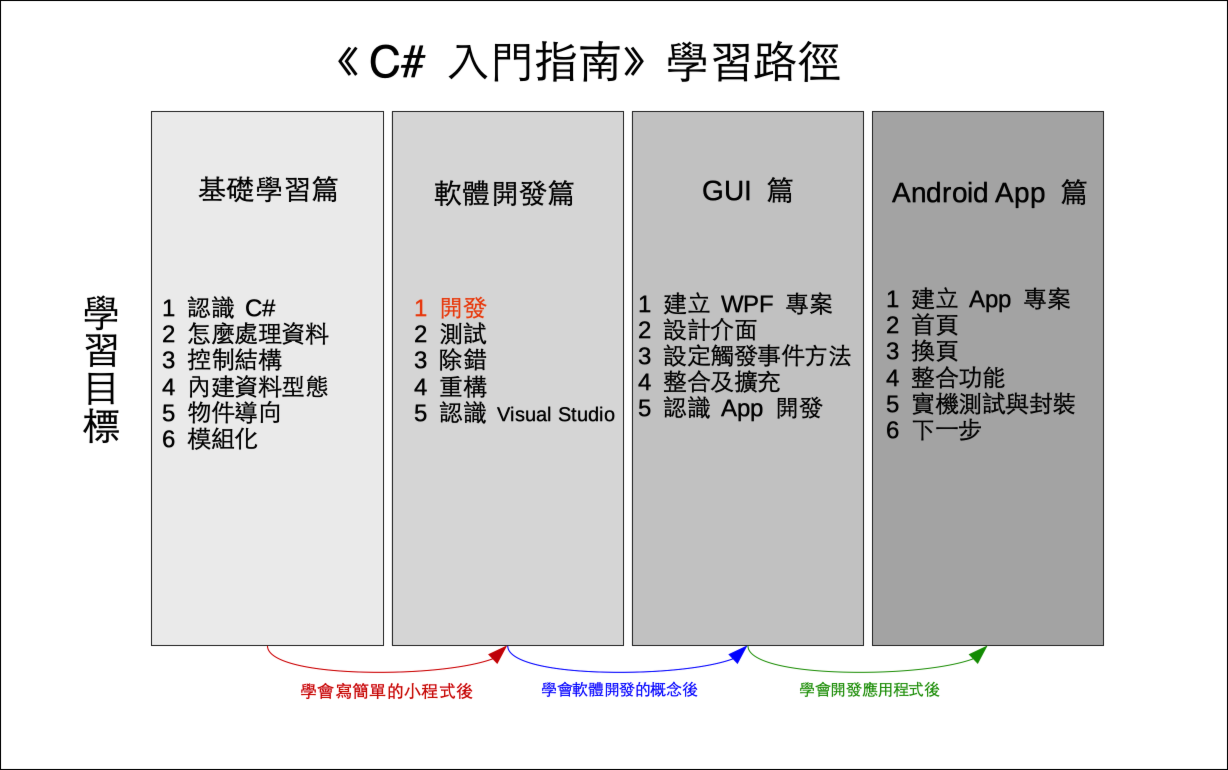# 單元 15 - 實作 SetCode()

～～學習進度表～～SetCode() 的工作就是建立密碼表，我們需要的密碼表就是一個攪亂順序的英文字母表

setCode()

 0 1 2 3 4 5 6 7 8 9 10 11 12 13 14 15 16 17 18 19 20 21 22 23 24 25 q z i r a j s b k t c l u d m v e n w f o x g p y h

``````y = a * x + b
m = y % n
r = m + diff``````

ab 預定是 09 之間的隨機整數，要取得隨機整數的話， .NET 有程式可用，倒是現在先不用急著一次到位，我們先把 a 設定成 3b 設定成 5 測試看看好了，如此 SetCode() 的實作如下

``````// 建立密碼表
public void SetCode()
{
// 宣告變數
int a, b, x, y, m;
char c = 'a';
// a 、 b 初始化
a = 3;
b = 5;
code = "";
// 建立密碼表的迴圈
for (int i = 0; i < 26; i++)
{
x = c;
y = x * a + b;
m = y % 26;
code += Convert.ToChar(m + 97);
c++;
}
}``````

code 欄位的初值設定為空字串 (empty string)

``code = "";``

``char c = 'a';``

for 迴圈中的 i 則是記錄迴圈 (loop) 進行的次數

``for (int i = 0; i < 26; i++)``

``````// 建立密碼表的迴圈
for (int i = 0; i < 26; i++)
{
x = c;
y = x * a + b;
m = y % 26;
code += Convert.ToChar(m + 97);
c++;
}``````

``x = c;``

``c++;``

``code += Convert.ToChar(m + 97);``

``````using System;

namespace EncryptDemo02
{
class EncryptDemo02
{
// 宣告密碼表欄位
public string code;

// 建構子，呼叫建立密碼表的 SetCode() 方法
public EncryptDemo02()
{
SetCode();
}

// 建立密碼表
public void SetCode()
{
// 宣告變數
int a, b, x, y, m;
char c = 'a';
// a 、 b 初始化
a = 3;
b = 5;
code = "";
// 建立密碼表的迴圈
for (int i = 0; i < 26; i++)
{
x = c;
y = x * a + b;
m = y % 26;
code += Convert.ToChar(m + 97);
c++;
}
}

// 編碼方法
public string ToEncode(string s)
{
return s;
}

// 解碼方法
public string ToDecode(string s)
{
return s;
}
}

class Program
{
static void Main(string[] args)
{
// 建立密碼物件
EncryptDemo02 e = new EncryptDemo02();
// 印出密碼表
Console.WriteLine(e.code);
}
}
}

//《程式語言教學誌》的範例程式
// http://kaiching.org/
// 專案：EncryptDemo02
// 檔名：Program.cs
// 功能：示範利用 C# 設計 Encrypt 類別
// 作者：張凱慶``````1. C# 程式設計手冊 | Microsoft Docs - 類別

1. 實作 SetCode() 是先將公式中的 a 設成 3b 設成 5 ，然後用迴圈利用兩個控制變數 ic 逐一計算每個字元。
2. 字元型態就是範圍較小的整數，因此可直接進行算術運算。
3. 計算完利用 SystemConvertToChar() 方法，將整數直接轉換成字元，再用 += 將字元附加到 code 欄位之後。

1. 為什麼不把建立對換表格直接寫在建構子裡就好了？
2. 為什麼數字要用 ConvertToChar() 方法轉轉換成字元，才可以用 += 附加到字串物件的最後？

1. 承接上一個單元的猜數字遊戲，另建立一個專案 Exercise1501 ，在 Program 類別的 Main() 方法接受使用者輸入，加入判斷使用者是否猜對的邏輯及提示訊息。
2. 承上題，另建立一個專案 Exercise1502 ，將 answer 改為字串。